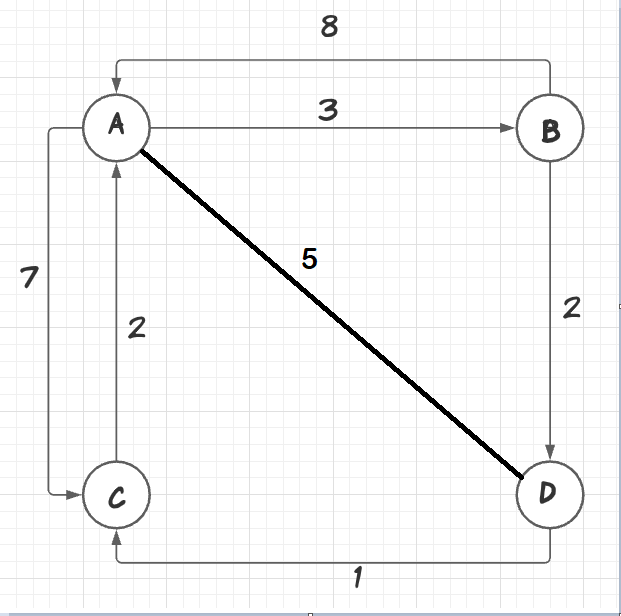Related Tags

communitycreator

# How to implement Floyd-Warshall algorithm in C by Greedy Method

S Sesha Savanth

## Floyd-Warshall

The Floyd-Warshall algorithm is used to find all pairs to the shortest path. This algorithm is used to find the shortest path between every pair of vertices in a given edge graph.

Let G = (V,E) be a directed graph with n vertices. Let cost be a cost adjacency matrix for G such that cost(i,i) = 0, 1<=i<=n.

Cost(i,j) = length or cost of edge (i,j), if(i,j) ∈ E(G) and cost(i,j)= ∞ if (i,j) ∉ E(G)

All-pairs shortest path problems is used to determine a matrix A such that A(i,j) is the length of a shortest path from i to j.

If k is the highest intermediate vertex between i to j path, then the i to k path will be the shortest path in graph G going through no vertex with an index greater than k-1. Similarly, the k to j path is the shortest path in graph G going through no vertex with index greater than k-1.

First, we need to find highest intermediate vertex k. Then, we need to find the two shortest paths from i to k and k to j.

Then, we will use $A^{k}$(i,j) to represent the length of the shortest path from i to j going through no vertex with an index of greater than k. This will give us:

$A^{0}$(i,j)=cost(i,j)

If it goes through the highest intermediate vertex k, then:

$A^{k}$(i,j) = $A^{k-1}$(i,k)+$A^{k-1}$(k,j)

If it does not go through the highest intermediate vertex k, then the highest intermediate vertex is:

$A^{k}$(i,j) = $A^{k-1}$(i,j)

To get a recurrence for $A^{k}$(i,j), we need to combine:

$A^{k}$(i,j) =min{ $A^{k-1}$(i,j), $A^{k-1}$(i,k)+$A^{k-1}$(k,j)}, where k>=1

Example:Note: For a selected intermediate vertex, the path that belongs to that vertex remains the same.

This is for A0 Matrix

By taking the above matrix we can get the $A^{1}$ matrix:

$A^{1}$(2,3) = min{$A^{0}$(2,3),$A^{0}$(2,1)+$A^{0}$(1,3)}

• $A^{1}$(2,3) = min{2,8+∞} = 2

$A^{1}$(2,4) = min{$A^{0}$(2,4),$A^{0}$(2,1)+$A^{0}$(1,4)}

• $A^{1}$(2,4) = min{∞,8+7} = 15

$A^{1}$(3,2) = min{$A^{0}$(3,2),$A^{0}$(3,1)+$A^{0}$(1,2)}

• $A^{1}$(3,2) = min{∞,5+3} = 8

$A^{1}$(3,4) = min{$A^{0}$(4,3),$A^{0}$(3,1)+$A^{0}$(1,4)}

• $A^{1}$(3,4) = min{1,5+7} = 1

$A^{1}$(4,2) = min{$A^{0}$(4,2),$A^{0}$(4,1)+$A^{0}$(1,2)}

• $A^{1}$(4,2) = min{∞,2+3} = 2

$A^{1}$(4,3) = min{$A^{0}$(4,3),$A^{0}$(4,1)+$A^{0}$(1,3)}

• $A^{1}$(4,3) = min{∞,2+∞} = 2
This is for A1 Matrix

### Similarly

This is for A2 Matrix
This is for A3 Matrix
This is for A4 Matrix

## Algorithm

for(k=0;k<n;k++)
{
for(i=0;i<n;i++)
{
for(j=0;j<n;j++)
{
if(a[i][j]>a[i][k]+a[k][j])
{
a[i][j] = a[i][k]+a[k[j];
}
}
}
}

#include<stdio.h>
void floyd(int a, int n)
{
for(int k=0;k<n;k++)
{
for(int i=0;i<n;i++)
{
for(int j=0;j<n;j++)
{
if(a[i][j]>a[i][k]+a[k][j])
{
a[i][j]=a[i][k]+a[k][j];
}
}
}
}
printf("All Pairs Shortest Path is :\n");
for(int i=0;i<n;i++)
{
for(int j=0;j<n;j++)
{
printf("%d ",a[i][j]);
}
printf("\n");
}
}
int main()
{
int cost = {{0, 3, 999, 4}, {8, 0, 2, 999}, {5, 999, 0, 1}, {2, 999, 999, 0}};
int n = 4;

floyd(cost,n);
}

RELATED TAGS

communitycreator

CONTRIBUTOR

S Sesha Savanth
RELATED COURSES

View all Courses

Keep Exploring

Learn in-demand tech skills in half the time